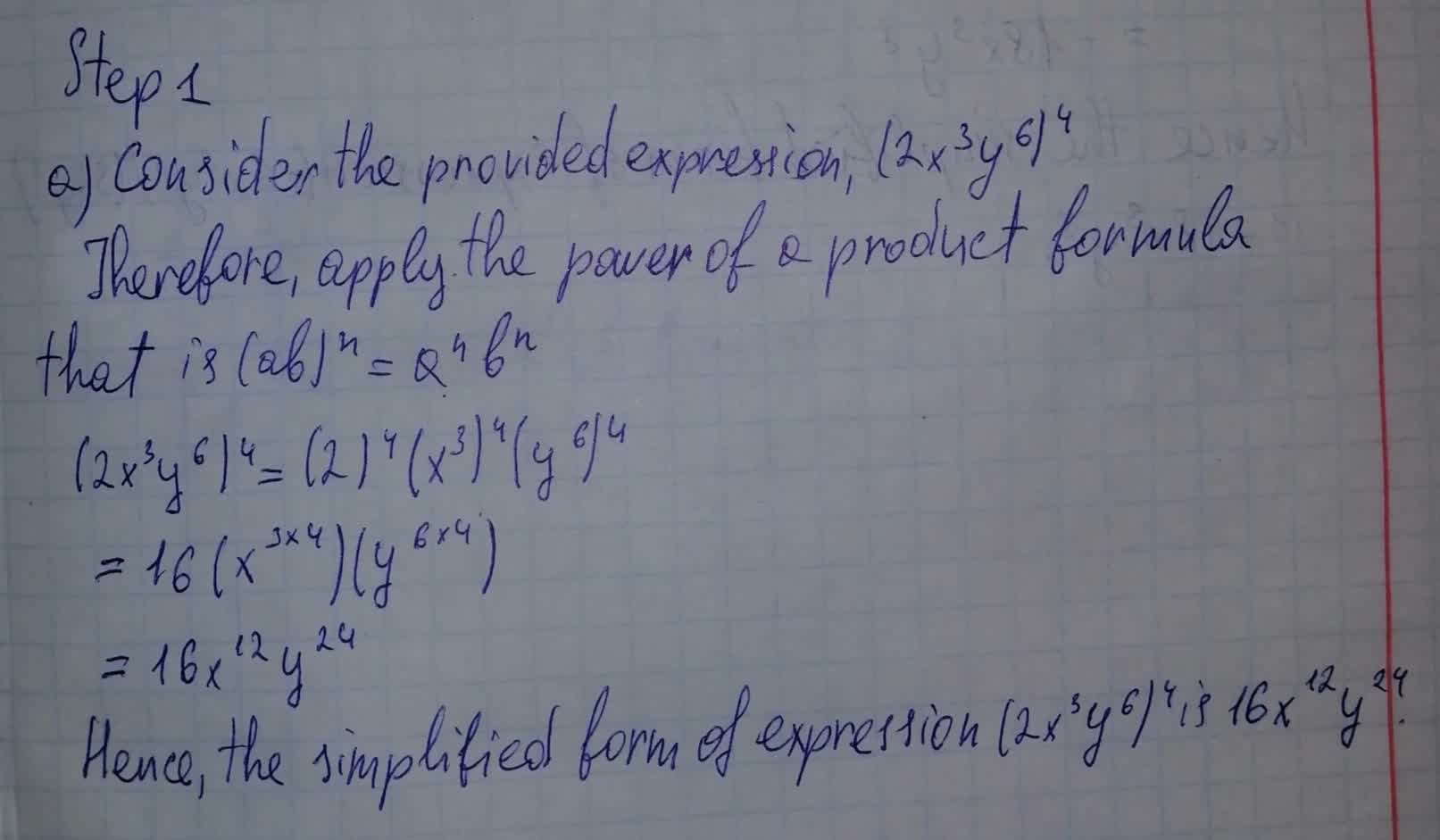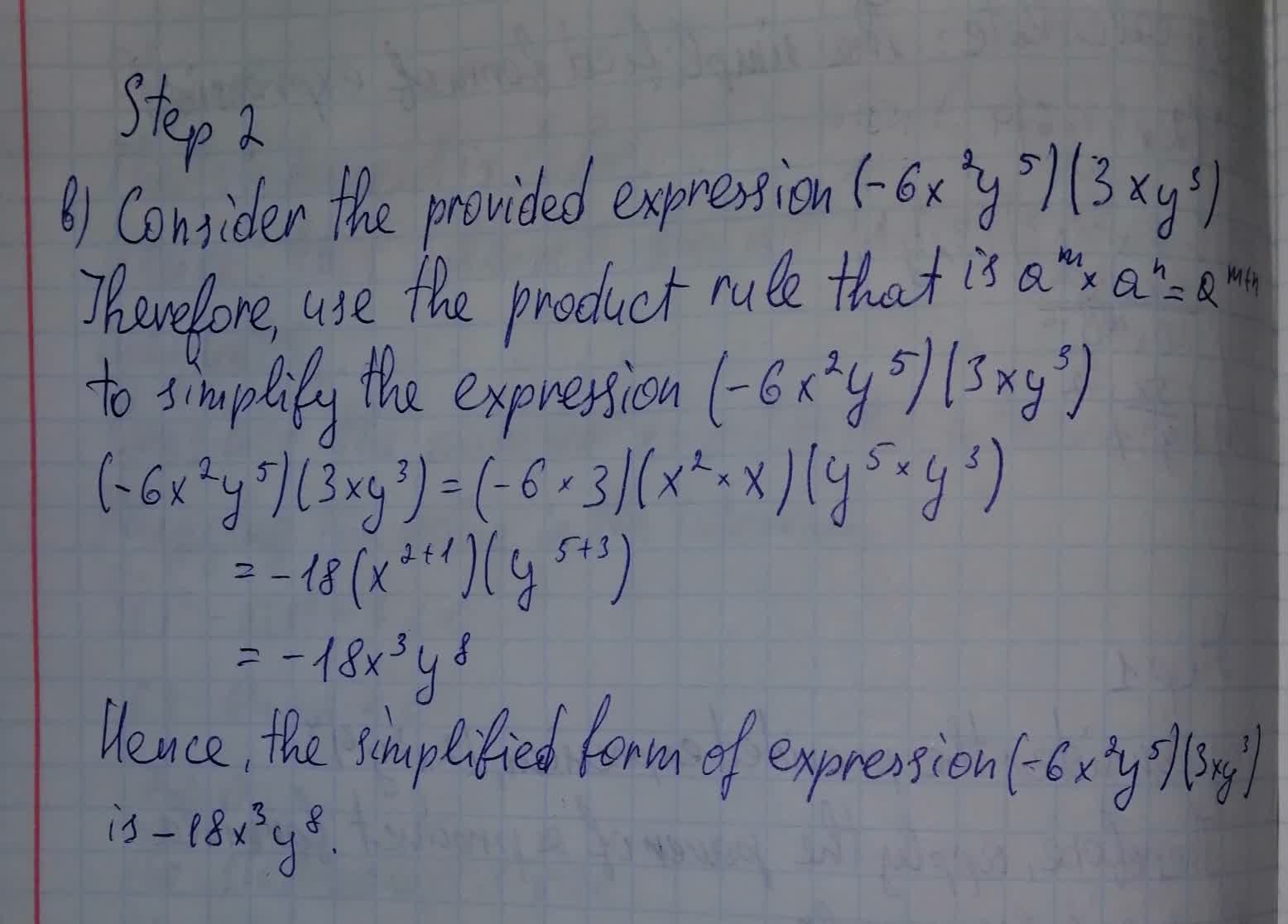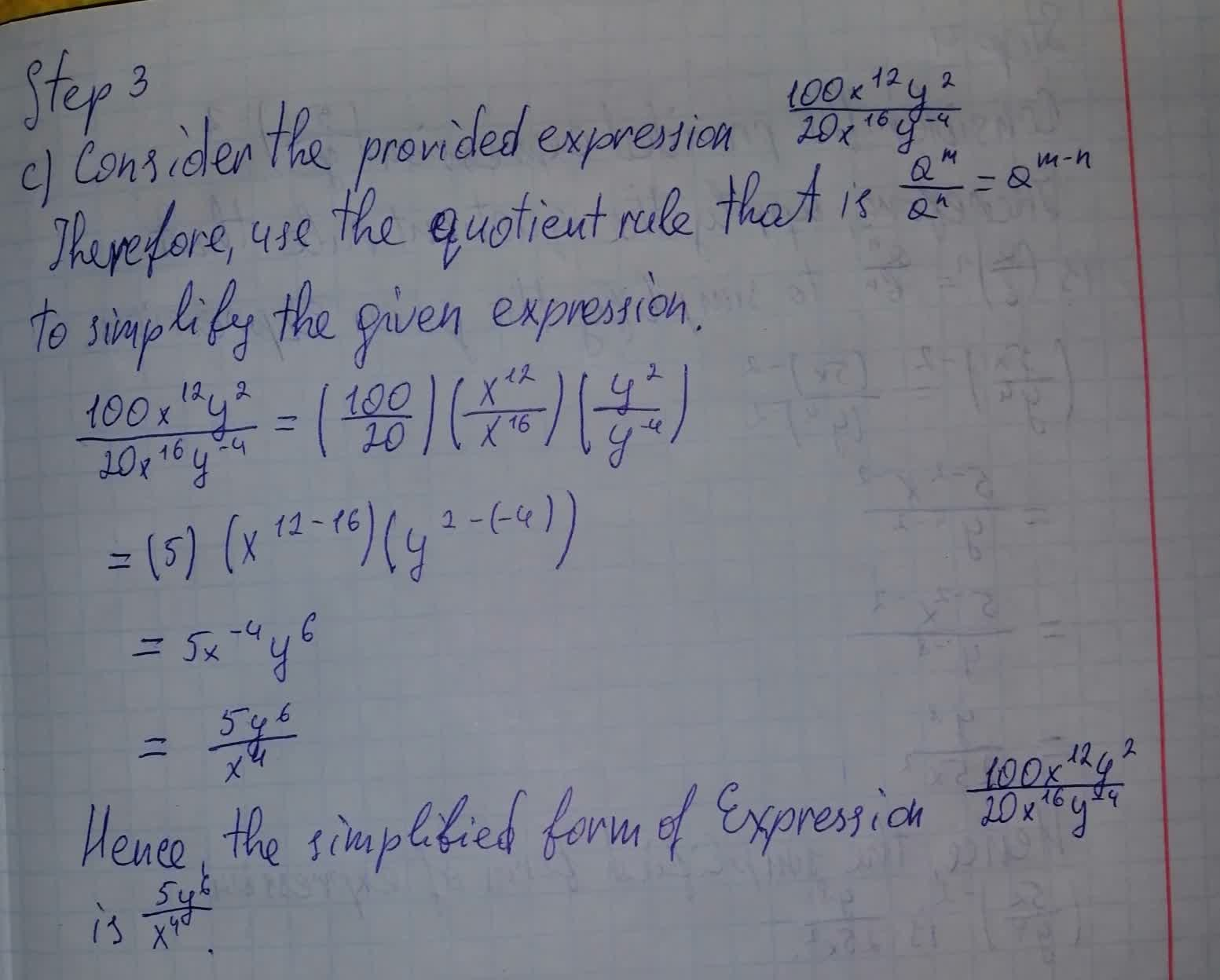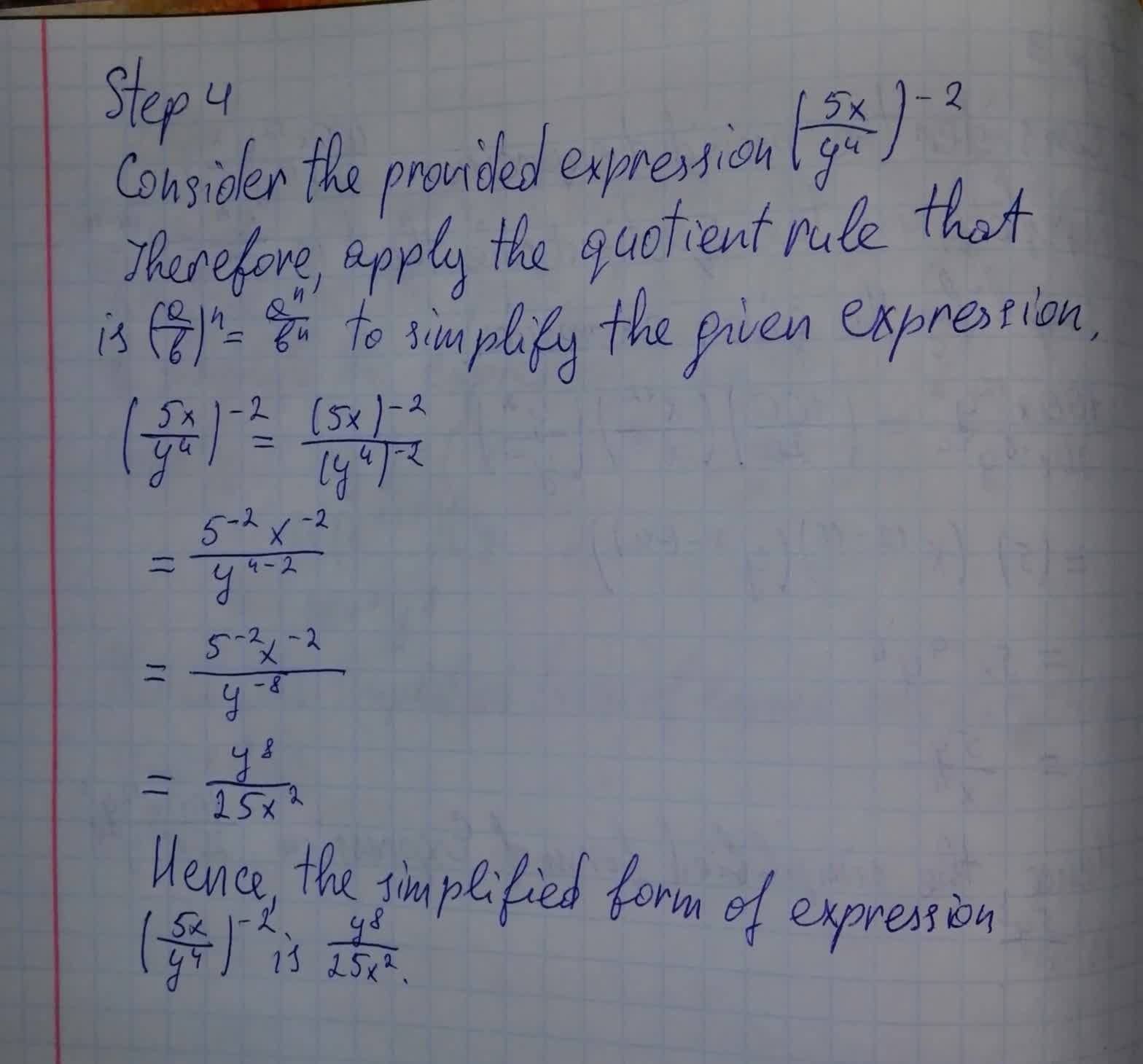To calculate: The simplified form of expression (2x^3 y^6)^4facas9 2021-08-09 Answered
To calculate: The simplified form of expression $$\displaystyle{\left({2}{x}^{{3}}{y}^{{6}}\right)}^{{4}}$$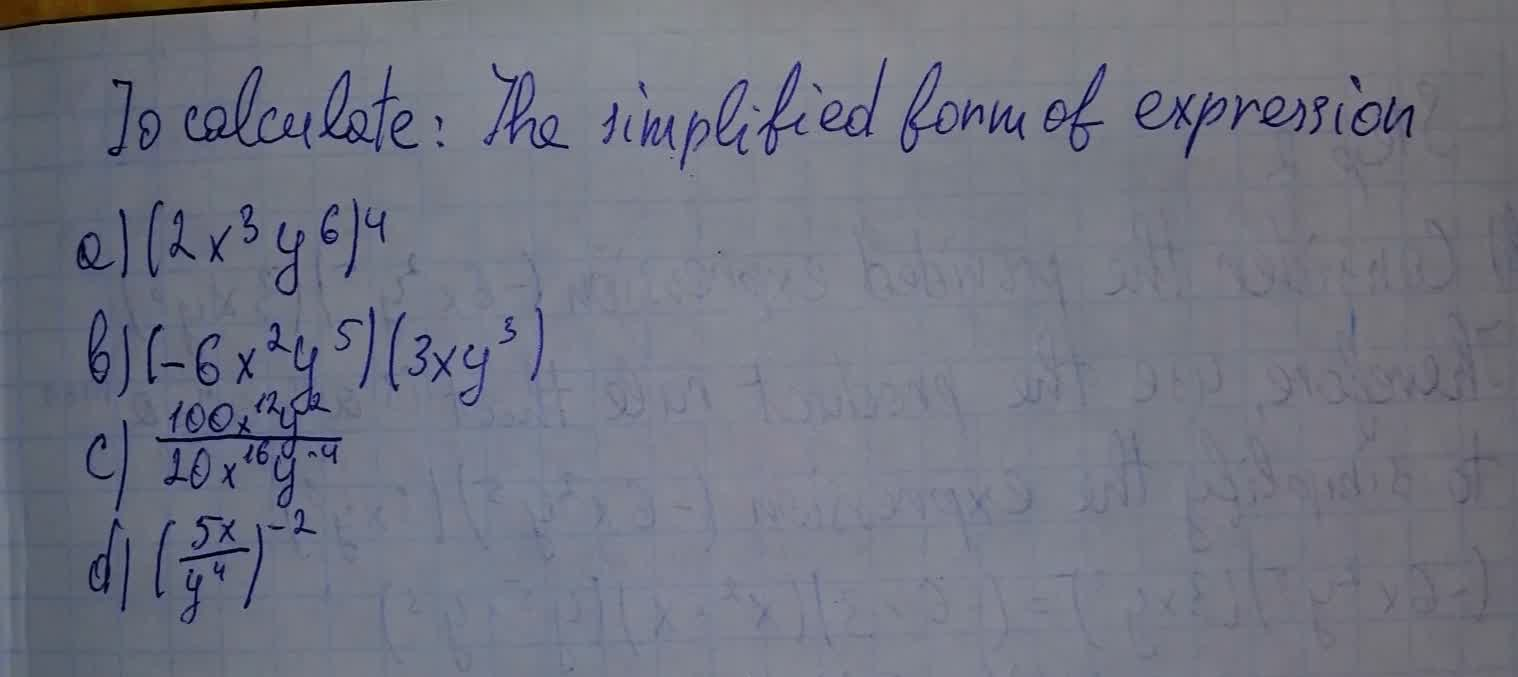• Questions are typically answered in as fast as 30 minutes

Solve your problem for the price of one coffee

• Math expert for every subject
• Pay only if we can solve itliannemdh

Calculation: